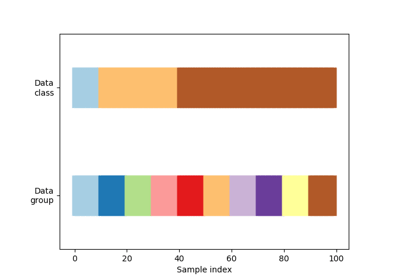/scikit-learn

# sklearn.model_selection.TimeSeriesSplit

`class sklearn.model_selection.TimeSeriesSplit(n_splits=’warn’, max_train_size=None)` [source]

Time Series cross-validator

Provides train/test indices to split time series data samples that are observed at fixed time intervals, in train/test sets. In each split, test indices must be higher than before, and thus shuffling in cross validator is inappropriate.

This cross-validation object is a variation of `KFold`. In the kth split, it returns first k folds as train set and the (k+1)th fold as test set.

Note that unlike standard cross-validation methods, successive training sets are supersets of those that come before them.

Read more in the User Guide.

Parameters: `n_splits : int, default=3` Number of splits. Must be at least 2. Changed in version 0.20: `n_splits` default value will change from 3 to 5 in v0.22. `max_train_size : int, optional` Maximum size for a single training set.

#### Notes

The training set has size ```i * n_samples // (n_splits + 1) + n_samples % (n_splits + 1)``` in the ```i``th split, with a test set of size ``n_samples//(n_splits + 1)```, where `n_samples` is the number of samples.

#### Examples

```>>> from sklearn.model_selection import TimeSeriesSplit
>>> X = np.array([[1, 2], [3, 4], [1, 2], [3, 4], [1, 2], [3, 4]])
>>> y = np.array([1, 2, 3, 4, 5, 6])
>>> tscv = TimeSeriesSplit(n_splits=5)
>>> print(tscv)
TimeSeriesSplit(max_train_size=None, n_splits=5)
>>> for train_index, test_index in tscv.split(X):
...    print("TRAIN:", train_index, "TEST:", test_index)
...    X_train, X_test = X[train_index], X[test_index]
...    y_train, y_test = y[train_index], y[test_index]
TRAIN:  TEST: 
TRAIN: [0 1] TEST: 
TRAIN: [0 1 2] TEST: 
TRAIN: [0 1 2 3] TEST: 
TRAIN: [0 1 2 3 4] TEST: 
```

#### Methods

 `get_n_splits`([X, y, groups]) Returns the number of splitting iterations in the cross-validator `split`(X[, y, groups]) Generate indices to split data into training and test set.
`__init__(n_splits=’warn’, max_train_size=None)` [source]
`get_n_splits(X=None, y=None, groups=None)` [source]

Returns the number of splitting iterations in the cross-validator

Parameters: `X : object` Always ignored, exists for compatibility. `y : object` Always ignored, exists for compatibility. `groups : object` Always ignored, exists for compatibility. `n_splits : int` Returns the number of splitting iterations in the cross-validator.
`split(X, y=None, groups=None)` [source]

Generate indices to split data into training and test set.

Parameters: `X : array-like, shape (n_samples, n_features)` Training data, where n_samples is the number of samples and n_features is the number of features. `y : array-like, shape (n_samples,)` Always ignored, exists for compatibility. `groups : array-like, with shape (n_samples,), optional` Always ignored, exists for compatibility. `train : ndarray` The training set indices for that split. `test : ndarray` The testing set indices for that split.

## Examples using `sklearn.model_selection.TimeSeriesSplit`Visualizing cross-validation behavior in scikit-learn